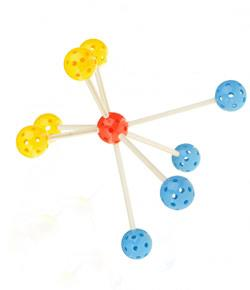# Unit 6 - Chemical Bonds Review

26 Questions | Total Attempts: 329SettingsIn chemistry, a bond is a lasting attraction between atoms that allows for the formation of chemical compounds, resulting from the electrostatic force of attraction between atoms with opposite charges. What else do you know about chemical bonds?

• 1.
Compounds have properties unlike those of the elements.
• A.

True

• B.

False

• 2.
In a chemical formula, a subscript tells how many atoms of an element are in a unit of a compound.
• A.

True

• B.

False

• 3.
A chemical bond occurs when atoms lose, gain, or share electrons.
• A.

True

• B.

False

• 4.
Because each noble gas has an outer energy level that is completely filled with electrons, these elements form chemical bonds easily.
• A.

True

• B.

False

• 5.
Compounds containing polyatomic ions can have both ionic and covalent bonds.
• A.

True

• B.

False

• 6.
A covalent bond is the force of attraction between the opposite charges of the ions in an ionic compound.
• A.

True

• B.

False

• 7.
Neutral particles formed as a result of covalent bonding are called molecules.
• A.

True

• B.

False

• 8.
An element that loses electrons when bonding with other atoms has a negative oxidation number.
• A.

True

• B.

False

• 9.
In a covalent bond, electrons are _____.
• A.

Lost

• B.

Gained

• C.

Shared

• D.

Destroyed

• 10.
When writing the formula of a compound, the symbol of the element with the positive oxidation number comes first.
• A.

True

• B.

False

• 11.
Chemical compounds are ____.
• A.

Always unstable

• B.

More stable than their individual elements

• C.

Less stable than their individual elements

• D.

Always stable

• 12.
Francium, which is the same chemical family as sodium, will carry what type of charge?
• A.

Both

• B.

Negative

• C.

Neutral

• D.

Positive

• 13.
What is the difference between carbon monoxide and carbon dioxide?
• A.

Carbon monoxide has only one oxygen atom

• B.

Carbon dioxide has two carbon atoms

• C.

Carbon monoxide has two carbon atoms

• D.

Carbon dioxide has only one oxygen atom

• 14.
The atom that contains the fewest electrons is ______.
• A.

Water

• B.

Hydrogen

• C.

Oxygen

• D.

Helium

• 15.
When elements form bonds, it changes their _____ properties.
• A.

Mass

• B.

Chemical and physical

• C.

Physical

• D.

Chemical

• 16.
Compounds that are hydrates contain what ingredient?
• A.

Nitrogen

• B.

Water

• C.

Hydrogen chloride

• D.

Chlorine

• 17.
What kind of charge does an ionic compound carry?
• A.

Unknown

• B.

Negative

• C.

Neutral

• D.

Positive

• 18.
The iodine ion (1-) carries what type of charge?
• A.

Positive

• B.

Neutral

• C.

Negative

• D.

Both

• 19.
Binary compounds are composed of ____ atoms.
• A.

One

• B.

Two

• C.

Many

• D.

Four

• 20.
NaCl is the chemical formula for what?
• A.

Sodium chloride

• B.

Sodium metal

• C.

Water

• D.

Chlorine gas

• 21.
For an ion, the oxidation number is ______.
• A.

Always negative

• B.

Zero

• C.

The same charge as the ion

• D.

One less than the charge on the ion

• 22.
In general, noble gases react with ____.
• A.

Nothing

• B.

Metals

• C.

Other noble gases

• D.

Water

• 23.
When an atom's outer energy levels are not filled completely, the atom _____.
• A.

Is a noble gas

• B.

Is very stable

• C.

Can gain, lose, or share electrons

• D.

Cannot form a compound

Related TopicsBack to top